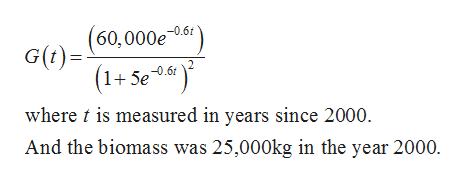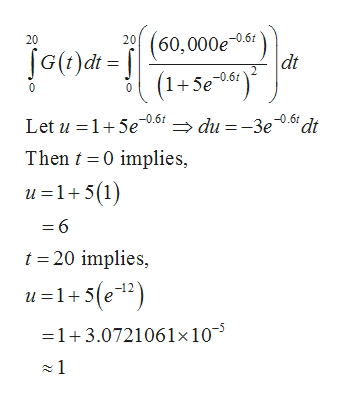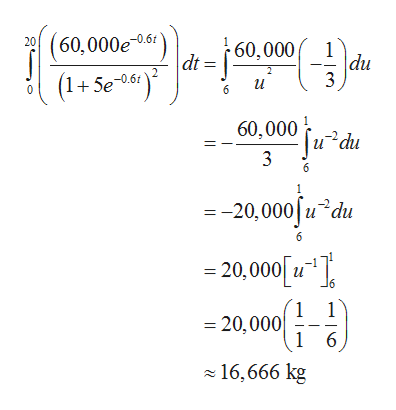# The rate of growth of the fish population was modeled by the equation G(t) = (60,000e^-0.6t)/(1+5e^-0.6t)^2, where t is measured in years since 2000 and G in kilograms per year. If the biomass was 25,000 kg in the year 2000, what is the predicted biomass for the year 2020?

Question
131 views

The rate of growth of the fish population was modeled by the equation G(t) = (60,000e^-0.6t)/(1+5e^-0.6t)^2, where t is measured in years since 2000 and G in kilograms per year. If the biomass was 25,000 kg in the year 2000, what is the predicted biomass for the year 2020?

check_circle

Step 1

Given:help_outlineImage Transcriptionclose-0.6f G(t)= (1+ Se*«) -0.61 where t is measured in years since 2000. And the biomass was 25,000kg in the year 2000. fullscreen
Step 2

The net change from 2000 to 2020 is,help_outlineImage Transcriptionclose60,000e-0.61 dt (1+5eo) 20 20 0.6f (1+5e061 Let u =1+5e -0.61 0.61 dt → du = -3e Then t =0 implies, u =1+ 5(1) = 6 t = 20 implies, u=1+5(e1?) =1+3.0721061×10¯³ z 1 fullscreen
Step 3

Therefore...help_outlineImage Transcriptionclose20 (60,000e -0s") -0.61 60,000 dt du 3 = + Se-0.61 60,000 iu²du 3 6. = -20,000|u du = 20,000[ 1" ), J6 = 20,000 6. 1 z 16,666 kg fullscreen

### Want to see the full answer?

See Solution

#### Want to see this answer and more?

Solutions are written by subject experts who are available 24/7. Questions are typically answered within 1 hour.*

See Solution
*Response times may vary by subject and question.
Tagged in

### Calculus